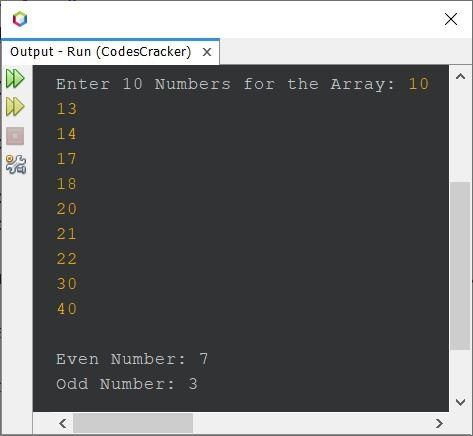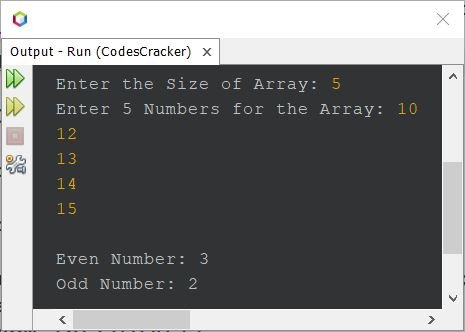# Java Program to Count Even and Odd Numbers in an Array

This article is created to cover a program in Java, to count and print the total number of even and odd numbers available in an array entered by user.

## Count Even and Odd Numbers in an Array of 10 Numbers

The question is, write a Java program to count even and odd numbers in an array of 10 elements. The 10 elements for the array must be received by user at run-time. Here is its answer:

```import java.util.Scanner;

public class CodesCracker
{
public static void main(String[] args)
{
int[] arr = new int;
Scanner s = new Scanner(System.in);

System.out.print("Enter 10 Numbers for the Array: ");
for(int i=0; i<10; i++)
arr[i] = s.nextInt();

int countEven=0, countOdd=0;
for(int i=0; i<10; i++)
{
if(arr[i]%2==0)
countEven++;
else
countOdd++;
}

System.out.println("\nEven Number: " +countEven);
System.out.println("Odd Number: " +countOdd);
}
}```

Here is its sample run with user input 10, 13, 14, 17, 18, 20, 21, 22, 30, 40 as 10 numbers:## Count Even and Odd Numbers in an Array of n Numbers

Since the program given above has a limitation, of only operating with 10 elements. Therefore I've modified that program with the program given below. This program allows user to define the size of array too, along with its elements:

```import java.util.Scanner;

public class CodesCracker
{
public static void main(String[] args)
{
Scanner s = new Scanner(System.in);

System.out.print("Enter the Size of Array: ");
int TOTAL = s.nextInt();
int[] arr = new int[TOTAL];
System.out.print("Enter " +TOTAL+" Numbers for the Array: ");
for(int i=0; i<TOTAL; i++)
arr[i] = s.nextInt();

int countEven=0, countOdd=0;
for(int i=0; i<TOTAL; i++)
{
if(arr[i]%2==0)
countEven++;
else
countOdd++;
}

System.out.println("\nEven Number: " +countEven);
System.out.println("Odd Number: " +countOdd);
}
}```

Here is its sample run with user input 5 as size of array, and 10, 12, 13, 14, 15 as its five elements:Java Online Test

« Previous Program Next Program »